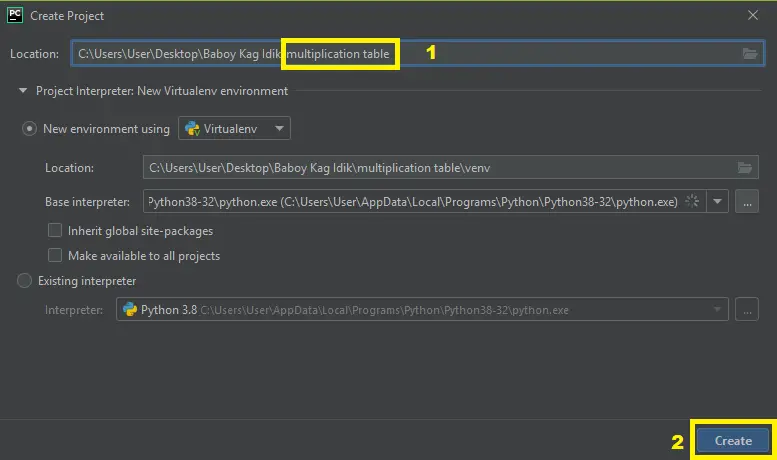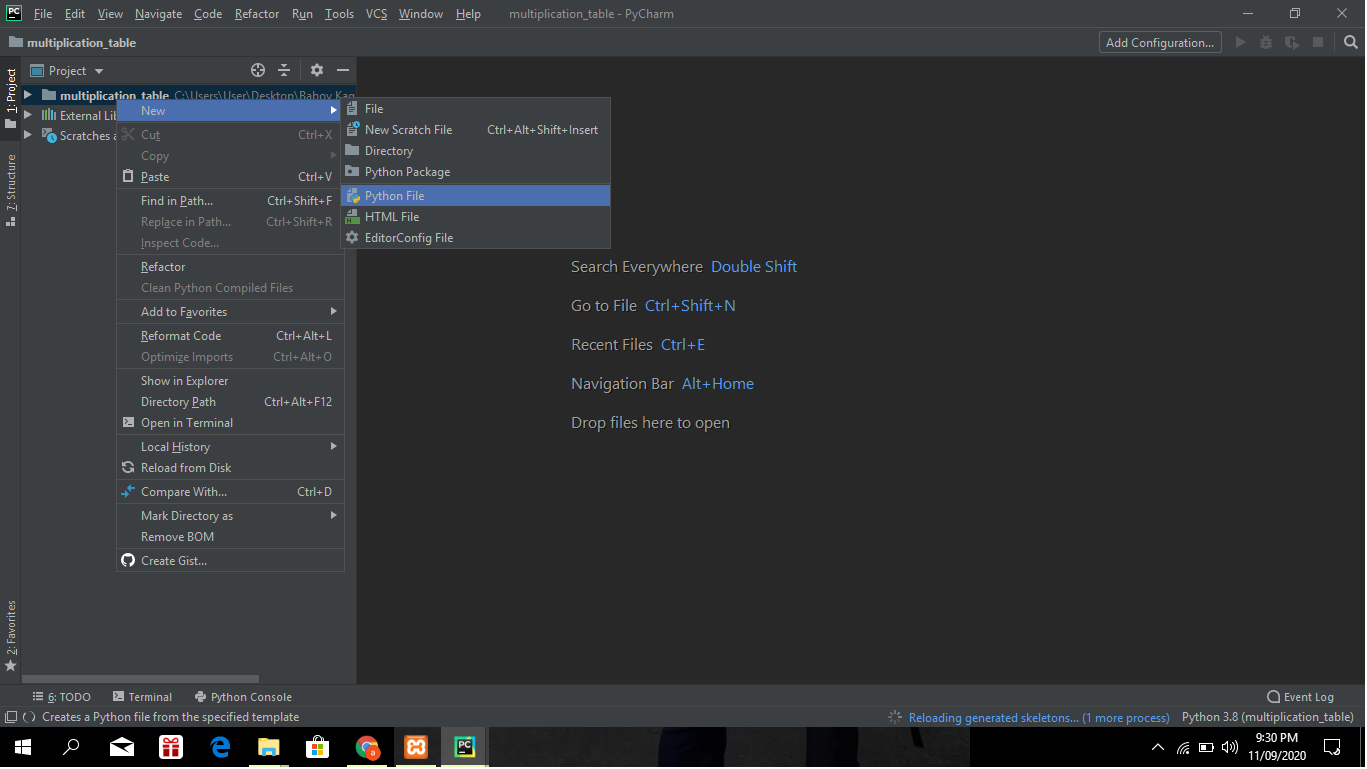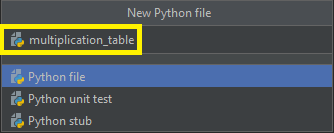# Multiplication Table In Python With Source Code

The Multiplication Table In Python is written in python programming language, In this tutorial you can learn on how to Display Multiplication Table In Python.

A Multiplication Table Python Program displays the multiplication table of variable num (from 1 to 10). In the program below, we have used the for loop to display the Multiplication Table Using Function of 12.

## Python Multiplication Table – Project Information’s

This article we have used the For Loop along with the range() function to iterate 10 times. The arguments inside the `range()` function are (1, 11). Meaning, greater than or equal to 1 and less than 11.

We have displayed the multiplication table of variable num (which is 12 in our case). You can change the value of num in the below program to test for other values.

To start creating a Multiplication Table In Python, make sure that you have PyCharm IDE installed in your computer.

## Steps on how to create a Multiplication Table In Python With Source Code

Time needed: 5 minutes.

These are the steps on how to create a Multiplication Table With Source Code

• Step 1: Create a project name.

First open Pycharm IDE and then create a “project name” after creating a project name click the “create” button.• Step 2: Create a python file.

Second after creating a project name, “right click” your project name and then click “new” after that click the “python file“.• Step 3: Name your python file.

Third after creating a python file, Name your python file after that click “enter“.• Step 4: The actual code.

You are free to copy the code given below and download the full source code below.

## Complete Source Code

``````# Multiplication table (from 1 to 10) in Python

num = 12

# To take input from the user

#int input/enter the number

# num = int(input("Display multiplication table of? "))

# Iterate 10 times from i = 1 to 10

for i in range(1, 11):

#print multiplication table

print(num, 'x', i, '=', num*i)``````

## Output

```12 x 1 = 12
12 x 2 = 24
12 x 3 = 36
12 x 4 = 48
12 x 5 = 60
12 x 6 = 72
12 x 7 = 84
12 x 8 = 96
12 x 9 = 108
12 x 10 = 120```